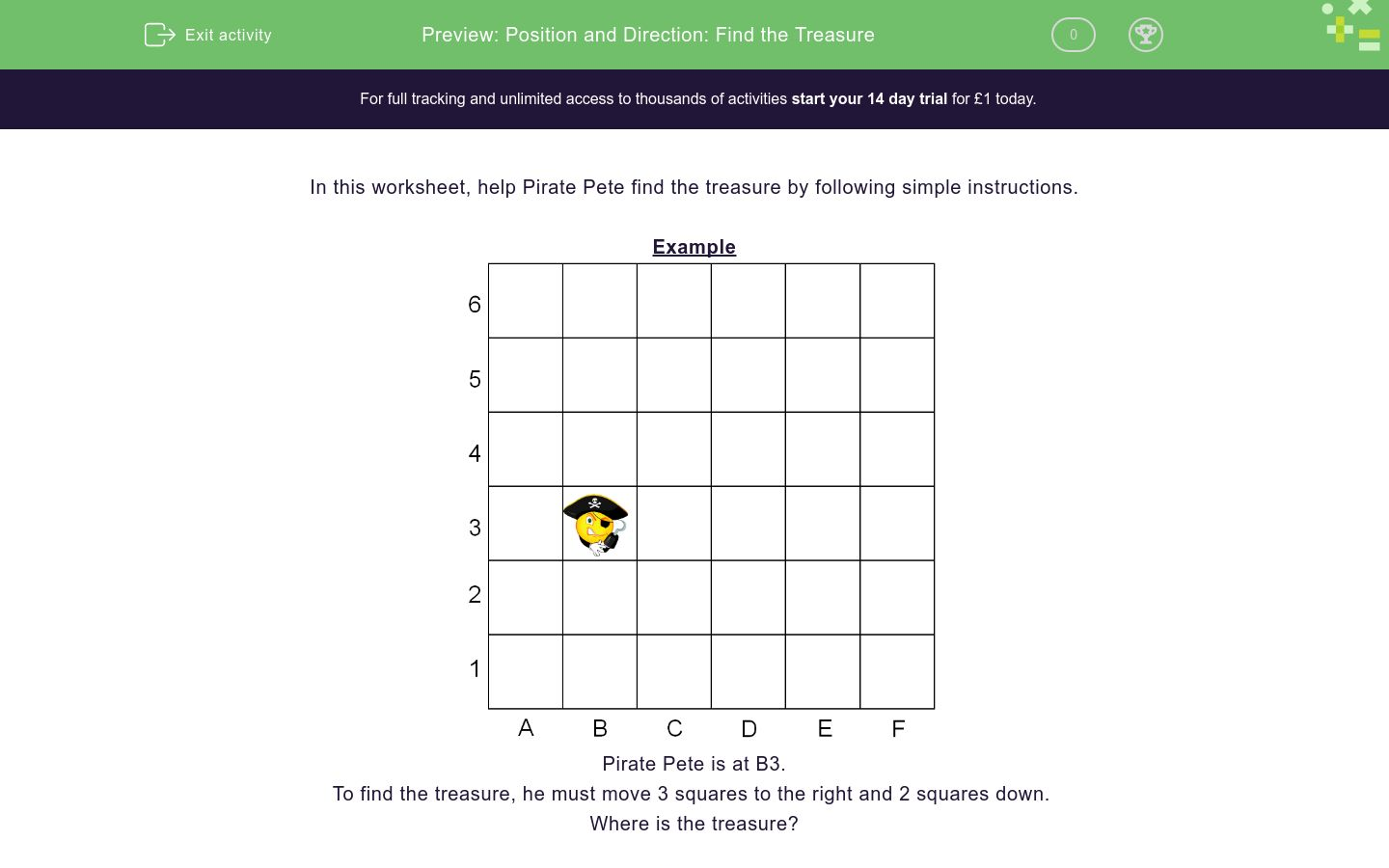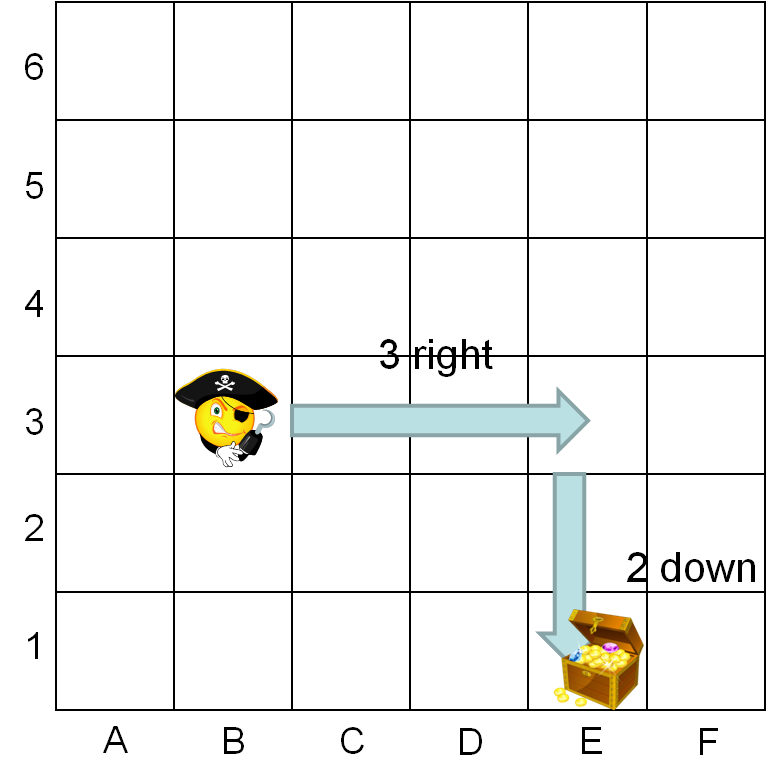# Position and Direction: Find the Treasure

In this worksheet, students use a grid and follow simple instructions relating to position, direction and movement to find the pirate's treasure!Key stage:  KS 2

Curriculum topic:   Geometry: Position and Direction

Curriculum subtopic:   Describe Movements of Position

Difficulty level:### QUESTION 1 of 10

In this worksheet, help Pirate Pete find the treasure by following simple instructions.

ExamplePirate Pete is at B3.

To find the treasure, he must move 3 squares to the right and 2 squares down.

Where is the treasure?The treasure is at E1

Always remember to write the letter first, followed by the number.

Pirate Pete is at B3.

To find the treasure, he must move 3 squares to the right and 2 squares up.

Where is the treasure?Don't forget to write the letter first and then the number.

Pirate Pete is at B3.

To find the treasure, he must move 2 squares to the right and 1 square up.

Where is the treasure?Don't forget to write the letter first and then the number.

Pirate Pete is at B3.

To find the treasure, he must move 1 square to the left and 3 squares up.

Where is the treasure?Don't forget to write the letter first and then the number.

Pirate Pete is at B3.

To find the treasure, he must move 2 squares down.

Where is the treasure?Don't forget to write the letter first and then the number.

Pirate Pete is at A1.

To find the treasure, he must move right 2 squares and 4 squares up.

Where is the treasure?Don't forget to write the letter first and then the number.

Pirate Pete is at A1.

To find the treasure, he must move right 5 squares and 2 squares up.

Where is the treasure?Don't forget to write the letter first and then the number.

Pirate Pete is at A1.

To find the treasure, he must move right 2 squares and 5 squares up.

Where is the treasure?Don't forget to write the letter first and then the number.

Where is Pirate Pete?

Where is the treasure?Don't forget to write the letter first (remember to write the letter in capitals) and then the number.

 Pirate Pete Treasure Position

Give Pirate Pete instructions to find the treasure.3 squares right and 2 down

4 squares right and 2 down

3 squares right and 1 down

Give Pirate Pete instructions to find the treasure.3 squares right and 4 up

2 squares left and 3 up

3 squares left and 4 up

• Question 1

Pirate Pete is at B3.

To find the treasure, he must move 3 squares to the right and 2 squares up.

Where is the treasure?Don't forget to write the letter first and then the number.

E5
E 5
EDDIE SAYS
Move 3 squares to the right and 2 squares up to E5.
• Question 2

Pirate Pete is at B3.

To find the treasure, he must move 2 squares to the right and 1 square up.

Where is the treasure?Don't forget to write the letter first and then the number.

D4
D 4
EDDIE SAYS
Move 2 squares to the right and 1 square up to D4.
• Question 3

Pirate Pete is at B3.

To find the treasure, he must move 1 square to the left and 3 squares up.

Where is the treasure?Don't forget to write the letter first and then the number.

A6
A 6
EDDIE SAYS
Move 1 square to the left and 3 squares up to A6.
• Question 4

Pirate Pete is at B3.

To find the treasure, he must move 2 squares down.

Where is the treasure?Don't forget to write the letter first and then the number.

B1
B 1
EDDIE SAYS
Move 2 squares down to B1.
• Question 5

Pirate Pete is at A1.

To find the treasure, he must move right 2 squares and 4 squares up.

Where is the treasure?Don't forget to write the letter first and then the number.

C5
C 5
EDDIE SAYS
Move right 2 squares and 4 squares up to C5.
• Question 6

Pirate Pete is at A1.

To find the treasure, he must move right 5 squares and 2 squares up.

Where is the treasure?Don't forget to write the letter first and then the number.

F3
F 3
EDDIE SAYS
Move right 5 squares and 2 squares up to F3.
• Question 7

Pirate Pete is at A1.

To find the treasure, he must move right 2 squares and 5 squares up.

Where is the treasure?Don't forget to write the letter first and then the number.

C6
C 6
EDDIE SAYS
Move right 2 squares and 5 squares up to C6
• Question 8

Where is Pirate Pete?

Where is the treasure?Don't forget to write the letter first (remember to write the letter in capitals) and then the number.

 Pirate Pete Treasure Position
EDDIE SAYS
Pirate Pete is at point B5, while the treasure is at point E4.
• Question 9

Give Pirate Pete instructions to find the treasure.3 squares right and 1 down
EDDIE SAYS
Move 3 squares to the right and 1 square down.
• Question 10

Give Pirate Pete instructions to find the treasure.2 squares left and 3 up
EDDIE SAYS
Move 2 squares left and 3 squares up.
---- OR ----

Sign up for a £1 trial so you can track and measure your child's progress on this activity.

### What is EdPlace?

We're your National Curriculum aligned online education content provider helping each child succeed in English, maths and science from year 1 to GCSE. With an EdPlace account you’ll be able to track and measure progress, helping each child achieve their best. We build confidence and attainment by personalising each child’s learning at a level that suits them.

Get started